# JP5617748B2 - Charger - Google Patents

## Info

Publication number
JP5617748B2
JP5617748B2 JP2011086052A JP2011086052A JP5617748B2 JP 5617748 B2 JP5617748 B2 JP 5617748B2 JP 2011086052 A JP2011086052 A JP 2011086052A JP 2011086052 A JP2011086052 A JP 2011086052A JP 5617748 B2 JP5617748 B2 JP 5617748B2
Authority
JP
Japan
Prior art keywords
voltage
ac
power
output
input
Prior art date
Legal status (The legal status is an assumption and is not a legal conclusion. Google has not performed a legal analysis and makes no representation as to the accuracy of the status listed.)
Active
Application number
JP2011086052A
Other languages
Japanese (ja)
Other versions
JP2012222951A (en
Inventor

Original Assignee

Priority date (The priority date is an assumption and is not a legal conclusion. Google has not performed a legal analysis and makes no representation as to the accuracy of the date listed.)
Filing date
Publication date
Application filed by 株式会社デンソー filed Critical 株式会社デンソー
Priority to JP2011086052A priority Critical patent/JP5617748B2/en
Publication of JP2012222951A publication Critical patent/JP2012222951A/en
Application granted granted Critical
Publication of JP5617748B2 publication Critical patent/JP5617748B2/en
Application status is Active legal-status Critical
Anticipated expiration legal-status Critical

## Images

•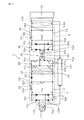•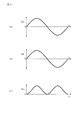•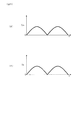•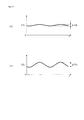•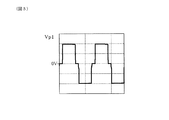•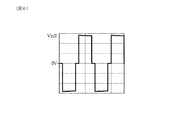•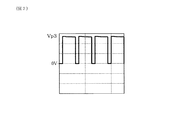•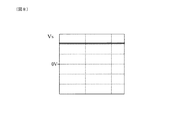•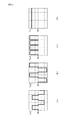•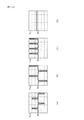•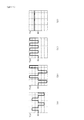•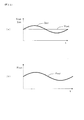•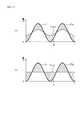•## Classifications

• HELECTRICITY
• H01BASIC ELECTRIC ELEMENTS
• H01MPROCESSES OR MEANS, e.g. BATTERIES, FOR THE DIRECT CONVERSION OF CHEMICAL ENERGY INTO ELECTRICAL ENERGY
• H01M10/00Secondary cells; Manufacture thereof
• H01M10/42Methods or arrangements for servicing or maintenance of secondary cells or secondary half-cells
• H01M10/44Methods for charging or discharging
• HELECTRICITY
• H02GENERATION; CONVERSION OR DISTRIBUTION OF ELECTRIC POWER
• H02JCIRCUIT ARRANGEMENTS OR SYSTEMS FOR SUPPLYING OR DISTRIBUTING ELECTRIC POWER; SYSTEMS FOR STORING ELECTRIC ENERGY
• H02J7/00Circuit arrangements for charging or depolarising batteries or for supplying loads from batteries
• H02J7/02Circuit arrangements for charging or depolarising batteries or for supplying loads from batteries for charging batteries from ac mains by converters
• H02J7/04Regulation of charging current or voltage

## Description

The present invention relates to a charging device for charging a secondary battery from an AC power supply.

A charging device for charging a secondary battery from an AC power supply includes an AC-DC converter that converts AC power into DC power. The AC-DC converter is provided with a smoothing capacitor for smoothing the rectified voltage after rectifying the AC input voltage.
This smoothing capacitor is generally required to have a sufficient capacity to sufficiently smooth the output voltage of the charging device. The reason is that if the smoothing capacitor does not have sufficient capacity, the output voltage will pulsate, and the secondary battery cannot be charged with a constant voltage and a constant current. It is because it ends.

Therefore, it is necessary to use a large-capacity capacitor as the smoothing capacitor, which makes it difficult to reduce the cost and size of the charging device.
Therefore, in the charging device described in Patent Document 1, the waveform of the AC input current is changed to a waveform including the fundamental wave component and its harmonic component by switching control using a switching element provided in the AC-DC converter. In addition, the output voltage waveform is controlled to have a predetermined pulsating flow. As a result, the capacity of the smoothing capacitor can be reduced.

JP 2010-88150 A

However, if the waveform of the AC input current is a waveform including the fundamental wave component and its harmonic component as described above, the power factor will be reduced. In addition, since the target waveform of the AC input current is not a sine wave, complicated control is required such that the control needs to be performed by a microcomputer. As a result, it becomes difficult to obtain an inexpensive charging device.
Further, it is difficult to control the output voltage to have a predetermined pulsating flow particularly when the capacity of the secondary battery is large. Therefore, in a charging device for charging a secondary battery having a large capacity such as a secondary battery mounted on an electric vehicle, a hybrid vehicle, or the like, it is not realistic to give a predetermined pulsating flow to the output voltage.

The present invention has been made in view of such problems, and an object of the present invention is to provide a charging device that can be easily reduced in size and cost without causing a reduction in power factor.

The present invention is a charging device for charging a secondary battery from an AC power source,
An AC-DC converter connected to the AC power source and a DC-DC converter connected to the secondary battery,
The AC-DC conversion unit generates an input rectification voltage by full-wave rectifying an AC input voltage to generate an input rectification voltage, and generates a DC voltage to be input to the DC-DC conversion unit by smoothing the input rectification voltage. And a smoothing capacitor that
The DC-DC converter includes a switching circuit that generates a pulse primary voltage from the DC voltage, a transformer that generates a pulse secondary voltage by transforming the pulse primary voltage, and full-wave rectifies the pulse secondary voltage. An output rectifier circuit that generates a pulse rectified voltage, and an output smoothing circuit that smoothes the pulse rectified voltage and generates output power for charging the secondary battery,
The switching circuit controls the output power to have a pulsating flow by adjusting the time ratio of the pulse primary voltage so that the waveform of the output power is synchronized with the waveform of the AC input power from the AC power supply. Has been
Further, the pulsating flow of the output power is configured to be generated by changing the output current while keeping the output voltage constant,
The charging device includes input detection means for detecting the AC input power, and output detection means for detecting the output power,
Based on the AC input power detected by the input detection means, a target output power that is the target output power is calculated,
The adjustment of the duty ratio of the pulse primary voltage is such that the waveform of the output power is synchronized with the waveform of the AC input power from the AC power supply based on the target output power and the output power detected by the output detection means. The charging device is configured to be performed as described above (claim 1).

The charging device is configured so that the output power has a pulsating flow and the waveform of the output power is synchronized with the waveform of the AC input power by controlling the switching circuit. Therefore, when the AC input power supplied from the AC power source is large, the output power is large, and when the AC input power is small, the output power is small. Therefore, it is possible to reduce the amount of power to be stored in the smoothing capacitor in the AC-DC conversion unit and the amount of power to be supplemented from the smoothing capacitor to the DC-DC conversion unit side. Therefore, the capacity of the smoothing capacitor can be reduced. As a result, the smoothing capacitor can be reduced in size and cost, and as a result, the charging device can be easily reduced in size and cost.

Moreover, since said control controls the waveform of output electric power, it does not control alternating current input current. Therefore, there is no possibility that the power factor decreases due to the waveform of the AC input current moving away from the sine wave.
Moreover, since the above control can be easily performed by adjusting the time ratio of the pulse primary voltage in the switching circuit, complicated control is not necessary.
Further, since the output voltage is not controlled, but the output power is controlled, the control is easy even when a secondary battery having a large capacity is charged.

Moreover, since the said charging device is provided with the said DC-DC conversion part, it is also possible to make the output voltage at the time of charging the said secondary battery high. Therefore, even if the output voltage fluctuates, the lower limit value can be prevented from falling below the voltage of the secondary battery (battery voltage). As a result, the output current can be prevented from becoming discontinuous, and the ripple current can be reduced. Thereby, it can prevent having a bad influence on the lifetime of a secondary battery.

As described above, according to the present invention, it is possible to provide a charging device that can be easily reduced in size and cost without causing a decrease in power factor.

1 is a circuit diagram of a charging device in Embodiment 1. FIG. In Example 1, (A) AC input voltage waveform diagram, (B) AC input current waveform diagram, and (C) AC input power waveform diagram. In Example 1, (A) Waveform diagram of input rectified voltage, (B) Waveform diagram of input rectified current. In Example 1, (A) A waveform diagram of a DC voltage when the capacity of the smoothing capacitor is relatively large, and (B) a waveform diagram of a DC voltage when the capacity of the smoothing capacitor is relatively small. FIG. 3 is a waveform diagram of a pulse primary voltage in the first embodiment. FIG. 3 is a waveform diagram of a pulse secondary voltage in the first embodiment. FIG. 3 is a waveform diagram of a pulse rectified voltage in the first embodiment. FIG. 3 is a waveform diagram of an output voltage in the first embodiment. (A) Waveform diagram of pulse primary voltage, (B) Waveform diagram of pulse secondary voltage, (C) Waveform diagram of pulse rectified voltage when the duty ratio of the pulse primary voltage in Example 1 is increased, (D) ) Waveform diagram of output voltage. (A) Waveform diagram of the pulse primary voltage, (B) Waveform diagram of the pulse secondary voltage, (C) Waveform diagram of the pulse rectified voltage when the duty ratio of the pulse primary voltage is lowered in Example 1. (D) ) Waveform diagram of output voltage. (A) Waveform diagram of the pulse primary voltage, (B) Waveform diagram of the pulse secondary voltage, (C) Waveform diagram of the pulse rectified voltage when the time ratio of the pulse primary voltage is set to the middle in Example 1. D) Waveform diagram of output voltage. In Example 1, (A) Waveform diagram of output voltage and output current, (B) Waveform diagram of output power. (A) Waveform diagram of AC input power and output power in Example 1, (B) Waveform diagram of AC input power and output power when the output power has no pulsating flow. FIG. 3 is a control flow diagram of a control unit in the first embodiment.

The charging device can be, for example, a charging device for charging a battery (secondary battery) mounted on a vehicle such as an electric vehicle or a plug-in hybrid vehicle from a commercial power source (AC power source).

Further, the charging apparatus, based on a difference between the output power and detected by the target output power and the output detecting means, to control the switching circuit so as to approximate the actual of the output power to the target output power It is preferable to be configured (claim 2). In this case, it becomes easy to control the waveform of the output power to a waveform close to ideal. Therefore, the capacity of the smoothing capacitor can be reduced more easily and reliably.

The AC-DC converter is a power factor correction circuit for bringing the waveform of the DC input current input to the DC-DC converter close to a sine wave between the input rectifier circuit and the smoothing capacitor. (Claim 3). In this case, the power factor of the charging device can be easily improved.

Example 1
A charging apparatus according to an embodiment of the present invention will be described with reference to FIGS.
The charging device 1 of this example is a charging device for charging the secondary battery 12 from the AC power source 11 as shown in FIG. 1, and includes an AC-DC converter 2 connected to the AC power source 11, The DC-DC conversion unit 3 is connected to the secondary battery 12.

The AC-DC converter 2 is an input rectifier circuit 21 that generates an input rectified voltage Va by full-wave rectifying the AC input voltage Vin, and a direct current that is input to the DC-DC converter 3 by smoothing the input rectified voltage Va. And a smoothing capacitor 23 for generating the voltage Vb.
The DC-DC converter 3 generates a switching circuit 31 that generates a pulse primary voltage Vp1 from a DC voltage Vb, a transformer 32 that transforms the pulse primary voltage Vp1 to generate a pulse secondary voltage Vp2, and a pulse secondary voltage Vp2. An output rectifier circuit 33 that generates a pulse rectified voltage Vp3 by full-wave rectification and an output smoothing circuit 34 that generates the output power Pout for smoothing the pulse rectified voltage Vp3 and charging the secondary battery 12 are provided. .
The switching circuit 31 adjusts the time ratio of the pulse primary voltage Vp1, thereby causing the output power Pout to have a pulsating flow as shown in FIG. 13A, and the waveform of the output power Pout is the alternating current from the AC power source 11. It is controlled so as to be synchronized with the waveform of the input power Pin.

As shown in FIG. 1, the AC-DC converter 2 brings the waveform of the AC input current Iin input to the AC-DC converter 2 between the input rectifier circuit 21 and the smoothing capacitor 23 closer to a sine wave. A PFC circuit (power factor correction circuit) 22 is provided.

The charging device 1 of this example is a charging device for charging a battery (secondary battery 11) mounted on a vehicle such as an electric vehicle or a plug-in hybrid vehicle from a commercial power source (AC power source 12).
The AC-DC converter 2 converts AC power input from an AC power source 11 such as a commercial power source into DC power. The input rectifier circuit 21 in the AC-DC converter 2 is a bridge-type rectifier circuit constituted by four diodes 21a, 21b, 21c, and 21d. The anode of the diode 21 a and the cathode of the diode 21 b are connected to one electrode in the AC power supply 11, and the anode of the diode 21 c and the cathode of the diode 21 d are connected to the other electrode in the AC power supply 11.

The cathodes of the diodes 21a and 21c are connected to the input high potential line H1, and the anodes of the diodes 21b and 21d are connected to the input low potential line L1.
With this configuration, the input rectifier circuit 21 performs full-wave rectification on the input AC input voltage Vin and generates the input rectified voltage Va between the input high potential line H1 and the input low potential line L1.

A smoothing capacitor 23 is connected between the input high potential line H1 and the input low potential line L1.
An inductor 221 is disposed on the input high potential line H <b> 1 between the input rectifier circuit 21 and one end of the smoothing capacitor 23. Further, a switching element 222 is connected between a terminal of the inductor 21 on the input high potential line H1 opposite to the input rectifier circuit 21 side and the input low potential line L1. As the switching element 222, for example, an IGBT (insulated gate bipolar transistor), a MOSFET (metal oxide field effect transistor), or the like can be used.
A diode 24 is disposed on the input high potential line H <b> 1 between one end of the switching element 222 and one end of the smoothing capacitor 23.

With such a circuit configuration, the AC-DC converter 2 forms a PFC circuit 22. In addition, the PFC circuit 22 is configured to turn on and off the switching element 222 by a general-purpose PFC-IC (not shown) or the like.

The AC-DC converter 2 configured as described above rectifies and smoothes the AC input voltage Vin from the AC power supply 11 and inputs the DC voltage Vb to the DC-DC converter 3. In addition, smoothing here is not complete smoothing, but means reducing the magnitude | size (voltage fluctuation width) of the pulse of a voltage waveform.

The DC-DC converter 3 includes a switching circuit 31 between the input high potential line H1 and the input low potential line L1. The switching circuit 31 is a full-bridge type switching circuit configured by four switching elements 31a, 31b, 31c, and 31d. The switching elements 31a and 31c have one end connected to the input high potential line H1 and the other end connected to one end of the other switching elements 31b and 31d. The switching elements 31b and 31d have the other end connected to the input low potential line L1.

A pair of terminals of the primary coil 321 in the transformer 32 is connected between the switching elements 31a and 31b connected to each other and between the switching elements 31c and 31d connected to each other. A pair of terminals of the secondary coil 322 of the transformer 32 is connected to the output rectifier circuit 33.

The output rectifier circuit 33 is a bridge-type rectifier circuit configured by four diodes 33a, 33b, 33c, and 33d. The anode of the diode 33a and the cathode of the diode 33b are connected to one terminal of the secondary coil 322 of the transformer 32, and the anode of the diode 33c and the cathode of the diode 33d are connected to the other terminal of the secondary coil 322. .
The cathodes of the diodes 33a and 33c are connected to the output high potential line H2, and the anodes of the diodes 33b and 33d are connected to the input low potential line L2.

An output smoothing circuit 34 is disposed between the output rectifier circuit 33 and the secondary battery 12.
The output smoothing circuit 34 includes an inductor 341 disposed on the output high potential line H2 and an output smoothing capacitor 342 connected between the output high potential line H2 and the output low potential line L2.
The full-bridge type DC-DC converter 3 configured in this way transforms the DC voltage Vb supplied from the AC-DC converter 2 (steps up in this example) and outputs it to the secondary battery 12. Electric power Pout is supplied. The secondary battery 12 can be, for example, a lithium ion battery, a nickel hydride battery, or the like.

Next, the operation of each part in the charging device 1 of this example will be described.
First, the AC input voltage Vin input from the AC power supply 11 to the AC-DC converter 2 has a sinusoidal waveform as shown in FIG. Here, in this example, the frequency of the AC input voltage Vin is 50 Hz.
The AC input current Iin input from the AC power supply 11 to the AC-DC converter 2 also has a similar sine wave waveform as shown in FIG. This is obtained as a result of being controlled by the PFC circuit 22 described above.
The AC input power Pin input from the AC power supply 11 to the AC-DC converter 2 is also a sine wave as shown in FIG. 2C, but the frequency is twice the frequency of the AC input voltage Vin. It becomes a certain 100 Hz.

The AC input voltage Vin having the waveform as described above is full-wave rectified in the input rectifier circuit 21 to become an input rectified voltage Va having a waveform as shown in FIG. The input rectified voltage Va has a frequency of 100 Hz, which is twice the frequency of the AC input voltage Vin. The waveform of the input rectified current Ia, which is a rectified current, also has a frequency (100 Hz) that is twice the AC input voltage Vin, as shown in FIG.

The input rectified voltage Va is smoothed by the smoothing capacitor 23 to become a DC voltage Vb. Here, if the capacity of the smoothing capacitor 23 is sufficiently increased, the smoothed DC voltage Vb waveform is a linear waveform with almost no pulses. However, when the capacity of the smoothing capacitor 23 is limited to some extent, the waveform of the DC voltage Vb becomes a pulsed waveform as shown in FIGS. 4 (A) and 4 (B). The frequency of this pulse is the same as the waveform of the input rectified voltage Va and is twice (100 Hz) the waveform of the AC input voltage Vin.
In this example, it is a premise that the capacity of the smoothing capacitor 23 is set to such a magnitude that a certain amount of pulsating current appears in the DC voltage Vb.

The fluctuation range ΔVb of the DC voltage Vb varies depending on the capacity of the smoothing capacitor 23. In other words, when the capacity of the smoothing capacitor 23 is relatively large, the fluctuation width ΔVb as shown in FIG. 4A becomes a relatively small voltage waveform, and when the capacity of the smoothing capacitor 23 is relatively small, FIG. A voltage waveform having a large fluctuation range ΔVb as shown in FIG.

A DC voltage Vb having such a pulsating flow is input to the DC-DC converter 3.
The DC voltage Vb is converted into the pulse primary voltage Vp1 in the switching circuit 31. In the switching circuit 31, the time for turning on only the switching element 31a and the switching element 31d, the time for turning off all the switching elements 31a to 31d, the time for turning on only the switching element 31b and the switching element 31c, and all the switching elements The pulse primary voltage Vp1 having a waveform as shown in FIG. 5 is generated by sequentially repeating the time for turning off 31a to 31d and applied to the primary coil 321 of the transformer 32. This switching control is performed at a high frequency, and in this example is performed at 50 kHz. The switching frequency is not limited to this, but is sufficiently higher than the frequency of the AC input voltage Vin.

When the switching control as described above is performed, a boosted pulse secondary voltage Vp2 is generated in the secondary coil 322 of the transformer 32 as shown in FIG. In this example, since the winding ratio of the transformer 32 is 1: 1.5, the pulse secondary voltage Vp2 is boosted to 1.5 times the pulse primary voltage Vp1.

This pulse secondary voltage Vp2 is full-wave rectified in the output rectifier circuit 33 and becomes a rectangular wave pulse rectified voltage Vp3 having a double frequency (100 kHz) as shown in FIG. The rectangular wave pulse rectified voltage Vp3 becomes the output voltage Vout smoothed by the output smoothing circuit 34. Along with this, the output current Iout flowing to the secondary battery 12 is also smoothed.

In the graphs shown in FIGS. 5 to 11, the vertical axis represents voltage and the horizontal axis represents time. Further, the scales (intervals between broken lines adjacent to the left and right) shown by vertical broken lines in FIGS. 5 to 11 indicate a certain time in all of these drawings. Moreover, the scale shown by the horizontal broken lines in FIGS. 5 to 11 (the interval between the broken lines adjacent to each other in the vertical direction) indicates a constant voltage value in all of these drawings. Moreover, the scale shown by the horizontal broken line in FIGS. 5 to 11 (the interval between the broken lines adjacent to each other in the vertical direction) indicates a constant current value in all of these drawings.

The output power Pout can be changed by changing the output current Iout. Although Pout = Vout × Iout, in this example, since the capacity of the secondary battery 12 is large, the output voltage Vout is constant. That is, since it is difficult to give an appropriate pulsating flow to the charging voltage to the secondary battery 12, the output power Pout is changed by changing the output current Iout.

To change the output current Iout, the time ratio (duty) of the pulse rectified voltage Vp3 is changed. Here, in explaining the fluctuation of the output current Iout due to the fluctuation of the time ratio of the pulse rectified voltage Vp3, the voltage after being smoothed by the output smoothing circuit 34 will be explained. That is, in the charging device 1 of this example, since the capacity of the secondary battery 12 is large, the output voltage Vout that is actually output to the secondary battery 12 does not vary. Therefore, a virtual circuit in which a resistor is connected instead of the secondary battery 12 in the circuit configuration shown in FIG. In this virtual circuit, when the time ratio (duty) of the pulse rectified voltage Vp3 is varied, the voltage applied to both ends of the output smoothing capacitor 342 (this is assumed to be the virtual voltage Vs) varies.

However, as shown in FIG. 12A, the actual output voltage Vout does not change as described above, and the output current Iout changes. That is, when the virtual voltage Vs is high, the output current Iout is large, and when the virtual voltage Vs is low, the output current Iout is small. Therefore, in order to change the output current Iout appropriately, it is only necessary to change the time ratio so that the virtual voltage Vs changes appropriately.

In order to increase the virtual voltage Vs, the time ratio (duty) of the pulse rectified voltage Vp3 may be increased, and in order to decrease the virtual voltage Vs, the time ratio of the pulse rectified voltage Vp3 may be decreased. Therefore, the virtual voltage Vs can be increased by increasing the time ratio of the pulse primary voltage Vp1, and the virtual voltage Vs can be decreased by decreasing the time ratio of the pulse primary voltage Vp1. This can be easily performed by on / off control of the switching elements 33a, 33b, 33c, and 33d in the output rectifier circuit 33.

That is, when increasing the virtual voltage Vs, the time ratio of the pulse primary voltage Vp1 is increased as shown in FIG. Accordingly, the time ratio of the pulse secondary voltage Vp2 increases as shown in FIG. 9B, and the time ratio of the pulse rectified voltage Vp3 increases as shown in FIG. 9C. Then, the virtual voltage Vs obtained by smoothing the pulse rectified voltage Vp3 having a high time ratio becomes a high voltage as shown in FIG. 9D.

On the contrary, when the virtual voltage Vs is lowered, the time ratio of the pulse primary voltage Vp1 is lowered as shown in FIG. Accordingly, the time ratio of the pulse secondary voltage Vp2 is lowered as shown in FIG. 10B, and the time ratio of the pulse rectified voltage Vp3 is also lowered as shown in FIG. 10C. Then, the virtual voltage Vs obtained by smoothing the pulse rectified voltage Vp3 having a low duty ratio becomes a low voltage as shown in FIG.

When the virtual voltage Vs is set to an intermediate voltage between the above two cases, the time ratio of the pulse primary voltage Vp1 is set to the intermediate between the above two cases as shown in FIG. As a result, the time ratio between the pulse secondary voltage Vp2 and the pulse rectified voltage Vp3 becomes an intermediate value as shown in FIGS. 11B and 11C, and the virtual voltage Vs is also set to an intermediate voltage as shown in FIG. Become.

As described above, the virtual voltage Vs can be easily adjusted by adjusting the duty ratio of the pulse primary voltage Vp1 under the control of the switching circuit 31, and the output current Iout can be adjusted as shown in FIG. it can. As a result, the output power Pout can be adjusted as shown in FIG. Therefore, it is possible to easily give a desired pulse to the output power Pout by appropriately controlling the fluctuation of the time ratio of the pulse primary voltage Vp1.

Therefore, in this example, by appropriately controlling the switching circuit 31, a desired pulse is given to the output current Iout, and an appropriate pulse is given to the output power Pout as shown by the curve Pout in FIG. Specifically, the waveform of the output power Pout is synchronized with the waveform of the AC input power Pin. That is, the frequency of the output power Pout is matched with the frequency of the AC input power Pin, and the output power Pout is high when the AC input power Pin is high, and the output power Pout is low when the AC input power Pin is low. . Further, the average value of the output power Pout is made to substantially match the average value of the AC input power Pin. However, the average value of the output power Pout has to be lower due to the charger efficiency.

A method for appropriately controlling the output power Pout will be described below.
As shown in FIG. 1, the charging device 1 of this example includes an input detection unit 131 that detects AC input power Pin, an output detection unit 132 that detects output power Pout, and a control unit 133 that controls the switching circuit 31. I have.

The control unit 133 calculates a target output power P3, which is a target output power, based on the AC input power Pin detected by the input detection unit 131. Then, the difference between the target output power P3 and the output power P2 detected by the output detection means 132 is calculated. Based on this difference, the switching circuit 31 is controlled to bring the actual output power Pout closer to the target output power P3.

An example of the control procedure will be described with reference to the flowchart shown in FIG.
First, a target power base P1 that serves as an axis of fluctuation of the target output power waveform (that is, an average value of the target output power) is set (step S1).
Next, the AC input power Pin is detected by the input detection means 131 (step S2).
Next, the target output power P3 is set using the target power base P1 and the AC input power Pin (step S3). In other words, the target output power is P3,
P3 = P1 + (Pin × K1) −K2 (1)
To calculate P3. The time variation of the target output power P3 becomes the target output power waveform.

Next, the actual output power P2 is detected by the output detection means 132 (step S4).
Next, the difference between the target output power P3 and the actual output power P2 is calculated (step S5).
Based on this difference, an appropriate value of the time ratio of the pulse primary voltage Vp1 is calculated and corrected (step S6).
Then, the switching circuit 31 is controlled so that the time ratio of the pulse primary voltage Vp1 becomes the calculated time ratio (step S7).

Steps S1 to S7 are repeated at the same frequency as the frequency (100 kHz) of the pulse rectified voltage Vp3. Thereby, the waveform of the output power Pout is made an ideal waveform, and the amount of power to be stored in the smoothing capacitor 23 and the amount of power to be supplemented from the smoothing capacitor 23 are reduced as much as possible.

Note that K1 and K2 in the calculation formula (1) using the target output power described above for calculating P3 are the following coefficients, respectively.
That is, K1 is a coefficient related to the fluctuation range of the target output power P3, and K2 is a coefficient related to the average value of the target output power.

A specific method for setting K1 and K2 will be described below.
First, the maximum value of the target output power is 110 W, the minimum value is 90 W, and the target power base P1 is 100 W. The charger efficiency is 90%. At this time, the AC input power Pin of the AC power supply 11 is determined, and it is assumed that the maximum value of Pin is 222 W and the minimum value is 0 W. Since the target output power P3 is synchronized with the AC input power Pin, the timings of the maximum value and the minimum value coincide with each other.
Then, the condition when the target output power P3 is the maximum and the condition when the target output power P3 is the minimum are substituted into the above equation (1). As a result, the following set of simultaneous equations is obtained.

110 = 100 + (222 × K1) −K2 (2)
90 = 100 + (0 × K1) −K2 Expression (3)

Expression (2) is a relational expression when the target output power P3 is maximum, and Expression (3) is a relational expression when the target output power is minimum.
By solving these simultaneous equations, K1 = 0.09 and K2 = 10 W are obtained.

Therefore, in the above situation, the calculation formula (1) used for calculating the target output power P3 is
P3 = P1 + (Pin × 0.09) −10 Equation (4)
It will be said that. In addition, the unit of the left side and the right side of Formula (4) is [W] (watt).

Next, the function and effect of this example will be described.
The charging device 1 is configured so that the output power Pout has a pulsating flow and the waveform of the output power Pout is synchronized with the waveform of the AC input power Pin by the control of the switching circuit 31. Therefore, as shown in FIG. 13A, when the AC input power Pin supplied from the AC power supply 11 is large, the output power Pout is large, and when the AC input power Pin is small, the output power Pout is small. Therefore, it is possible to reduce the amount of power to be stored in the smoothing capacitor 23 in the AC-DC converter 2 and the amount of power to be replenished from the smoothing capacitor 23 to the DC-DC converter 3 side.

That is, the area of each region (shaded portion E) surrounded by the waveform of the AC input power Pin and the output power Pout in FIG. 13A is the amount of power to be stored in the smoothing capacitor 23 or from the smoothing capacitor 23 to DC. -Corresponds to the amount of power to be supplemented to the DC converter 3 side. This amount of electric power can be significantly reduced as compared with the case where the output electric power Pout is not pulsed as shown in FIG.

Therefore, the capacity of the smoothing capacitor 23 can be reduced. As a result, the smoothing capacitor 23 can be reduced in size and cost, and as a result, the charging device 1 can be easily reduced in size and cost.

Moreover, since the above control is for controlling the waveform of the output power Pout, it is not for controlling the AC input current Iin. Therefore, there is no possibility that the power factor is lowered due to the waveform of the AC input current Iin moving away from the sine wave.
Further, the above control can be easily performed by adjusting the time ratio of the pulse primary voltage Vp1 in the switching circuit 31, so that complicated control is not necessary.
Moreover, since the output voltage Vout is not controlled but the output power Pout is controlled, the control is easy even when the secondary battery 12 having a large capacity is charged.

Moreover, since the charging device 1 includes the DC-DC conversion unit 3, the secondary side voltage of the DC-DC conversion unit 3, that is, the secondary battery 12 is charged compared to the primary side voltage (Ve) of the DC-DC conversion unit. It is also possible to increase the output voltage (virtual voltage Vs) during the process. Therefore, even if the primary voltage (Ve) of the DC-DC converter 3 fluctuates and its lower limit value is lower than the battery voltage, the secondary voltage of the DC-DC converter 3, that is, the output voltage (virtual voltage Vs). ) Can be prevented from falling below the voltage of the secondary battery 12 (battery voltage). As a result, the output current Iout can be prevented from becoming discontinuous, and the ripple current can be reduced. Thereby, it can prevent having a bad influence on the lifetime of the secondary battery 12. FIG.

As described above, according to this example, it is possible to provide a charging device that can be easily reduced in size and cost without causing a reduction in power factor.

DESCRIPTION OF SYMBOLS 1 Charging apparatus 11 AC power supply 12 Secondary battery 2 AC-DC conversion part 21 Input rectifier circuit 22 PFC circuit (power factor improvement circuit)
23 smoothing capacitor 3 DC-DC converter 31 switching circuit 32 transformer 33 output rectifier circuit 34 output smoother circuit Vin AC input voltage Va input rectified voltage Vb DC voltage Vp1 pulse primary voltage Vp2 pulse secondary voltage Vp3 pulse rectified voltage Vout output voltage Pin AC input power Pout Output power

## Claims (3)

1. A charging device for charging a secondary battery from an AC power source,
An AC-DC converter connected to the AC power source and a DC-DC converter connected to the secondary battery,
The AC-DC conversion unit generates an input rectification voltage by full-wave rectifying an AC input voltage to generate an input rectification voltage, and generates a DC voltage to be input to the DC-DC conversion unit by smoothing the input rectification voltage. And a smoothing capacitor that
The DC-DC converter includes a switching circuit that generates a pulse primary voltage from the DC voltage, a transformer that generates a pulse secondary voltage by transforming the pulse primary voltage, and full-wave rectifies the pulse secondary voltage. An output rectifier circuit that generates a pulse rectified voltage, and an output smoothing circuit that smoothes the pulse rectified voltage and generates output power for charging the secondary battery,
The switching circuit controls the output power to have a pulsating flow by adjusting the time ratio of the pulse primary voltage so that the waveform of the output power is synchronized with the waveform of the AC input power from the AC power supply. Has been
Further, the pulsating flow of the output power is configured to be generated by changing the output current while keeping the output voltage constant,
The charging device includes input detection means for detecting the AC input power, and output detection means for detecting the output power,
Based on the AC input power detected by the input detection means, a target output power that is the target output power is calculated,
The adjustment of the duty ratio of the pulse primary voltage is such that the waveform of the output power is synchronized with the waveform of the AC input power from the AC power supply based on the target output power and the output power detected by the output detection means. The charging device is configured to be performed as described above .
2. The charging device according to claim 1, wherein the switching circuit is configured to bring the actual output power closer to the target output power based on a difference between the target output power and the output power detected by the output detection unit. A charging device configured to control.
3.   3. The charging device according to claim 1, wherein the AC-DC conversion unit includes a power factor correction circuit configured to approximate the waveform of the AC input current input to the AC-DC conversion unit to a sine wave. A charging device characterized by that.
JP2011086052A 2011-04-08 2011-04-08 Charger Active JP5617748B2 (en)

## Priority Applications (1)

Application Number Priority Date Filing Date Title
JP2011086052A JP5617748B2 (en) 2011-04-08 2011-04-08 Charger

## Applications Claiming Priority (1)

Application Number Priority Date Filing Date Title
JP2011086052A JP5617748B2 (en) 2011-04-08 2011-04-08 Charger

## Publications (2)

Publication Number Publication Date
JP2012222951A JP2012222951A (en) 2012-11-12
JP5617748B2 true JP5617748B2 (en) 2014-11-05

# Family

## Family Applications (1)

Application Number Title Priority Date Filing Date
JP2011086052A Active JP5617748B2 (en) 2011-04-08 2011-04-08 Charger

## Country Status (1)

JP (1) JP5617748B2 (en)

## Families Citing this family (3)

* Cited by examiner, † Cited by third party
Publication number Priority date Publication date Assignee Title
JP2015144554A (en) 2013-12-24 2015-08-06 パナソニックＩｐマネジメント株式会社 Power conversion equipment
EP3282551B1 (en) * 2016-02-05 2019-08-14 Guangdong Oppo Mobile Telecommunications Corp., Ltd. Adapter and charging control method
KR101995942B1 (en) * 2017-07-26 2019-07-05 주식회사비엠테크 Managing system of smart plant through no power supplier using the magnetic field of high voltage cable

## Family Cites Families (5)

* Cited by examiner, † Cited by third party
Publication number Priority date Publication date Assignee Title
JPH05103430A (en) * 1991-10-07 1993-04-23 Murata Mfg Co Ltd Battery charging circuit
JPH07115736A (en) * 1993-10-15 1995-05-02 Hitachi Ltd Charging device for electric rolling stock
JPH0888906A (en) * 1994-09-14 1996-04-02 Hitachi Ltd Charger for electric railcar
JPH08107607A (en) * 1994-10-03 1996-04-23 Hitachi Ltd Charger for electric railcar battery
JP2009247101A (en) * 2008-03-31 2009-10-22 Tdk Corp Charger device

## Also Published As

Publication number Publication date
JP2012222951A (en) 2012-11-12

## Similar Documents

Publication Publication Date Title
US7453248B2 (en) Switching power supply device
CN101741244B (en) Method and apparatus to increase efficiency in a power factor correction circuit
US10355526B2 (en) Bi-directional inductive power transfer
US8004262B2 (en) Method and apparatus to control a power factor correction circuit
JP5104947B2 (en) Switching power supply
EP1575152B1 (en) Power factor correction circuits
US7919950B2 (en) Power factor correction converter
US8395366B2 (en) Power factor correction converter including input current detecting circuit
US20120092900A1 (en) Controller with punctuated switching control circuit
EP2458722B1 (en) LED driving apparatus
TWI533583B (en) A hysteretic-mode pulse frequency modulated (hm-pfm) resonant ac to dc converter
US20150048807A1 (en) Power Factor Correction Circuit and Method
US20110110127A1 (en) Power factor correction circuit
JP5136364B2 (en) Control method of power factor correction circuit
US8044643B1 (en) Power supply switching circuit for a halogen lamp
US8493757B2 (en) AC/DC converter with a PFC and a DC/DC converter
WO2011030640A1 (en) Pfc converter
US8320139B2 (en) Switching regulator with frequency limitation and method thereof
CN102638169A (en) Control circuit and control method of flyback convertor and alternating current-direct current power converting circuit applying control circuit of flyback convertor
US6711034B2 (en) DC-DC converter and a regulation method for this DC-DC converter
US8953348B2 (en) Switching power supply circuit and power factor controller
EP2321892B1 (en) Switched-mode power supply having self-optimizing efficiency
WO2013056356A1 (en) Ripple cancellation converter with high power factor
Jung et al. High efficiency bidirectional LLC resonant converter for 380V DC power distribution system using digital control scheme
JP6080345B2 (en) Switching power supply and AC waveform generation method in switching power supply

## Legal Events

Date Code Title Description
A621 Written request for application examination

Free format text: JAPANESE INTERMEDIATE CODE: A621

Effective date: 20130426

A977 Report on retrieval

Free format text: JAPANESE INTERMEDIATE CODE: A971007

Effective date: 20140227

A131 Notification of reasons for refusal

Free format text: JAPANESE INTERMEDIATE CODE: A131

Effective date: 20140311

A521 Written amendment

Free format text: JAPANESE INTERMEDIATE CODE: A523

Effective date: 20140423

TRDD Decision of grant or rejection written
A01 Written decision to grant a patent or to grant a registration (utility model)

Free format text: JAPANESE INTERMEDIATE CODE: A01

Effective date: 20140819

A61 First payment of annual fees (during grant procedure)

Free format text: JAPANESE INTERMEDIATE CODE: A61

Effective date: 20140901

R151 Written notification of patent or utility model registration

Ref document number: 5617748

Country of ref document: JP

Free format text: JAPANESE INTERMEDIATE CODE: R151

R250 Receipt of annual fees

Free format text: JAPANESE INTERMEDIATE CODE: R250

R250 Receipt of annual fees

Free format text: JAPANESE INTERMEDIATE CODE: R250

R250 Receipt of annual fees

Free format text: JAPANESE INTERMEDIATE CODE: R250